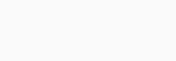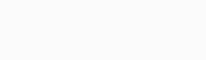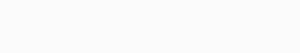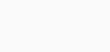# Understanding the charge in a capacitor

In a previous article on capacitors, they are known to store electrical energy on their plates in the form of an electrical charge. Today you’ll get to know the capacitance and charge in a capacitor.Conventionally, capacitors consist of two parallel conductive plates (usual metals) which are demarcated (separated) by an insulating material known as “dielectric”. so, whenever a voltage is subjected to these plates, an electrical current flows charging up one plate with a positive charge to the supply voltage. The other plates receive an equal and opposite negative charge.

This gives the capacitor the ability to store an electrical charge Q (units in Coulombs) of electrons. There is a potential difference, p.d between the plates of a capacitor when it is fully charged. Also, the larger the area of the plates and/or the smaller the distance between them is called separation. The greater will be the charge that the capacitor can hold and it will be its CAPACITANCE.

Furthermore, capacitor’s ability to store electrical charge, Q between their plates is proportional to the applied voltage, V for a capacitor of known capacitance in Farads. You should know that a capacitance C can never be negative but is always positive. The greater the applied voltage the greater the charge stored on the plates of the capacitor. Likewise, the smaller the applied voltage the smaller the charge. Thus, the actual charge Q on the plates of the capacitor can be calculated as:

Charge on a capacitor     Q = C x V

Where: Q (Charge, in coulombs) = C (Capacitance, in Farads) x V (Voltage, in Volts).

This same equation can be transposed and gives the following combinations:

Q = C x V              or C = Q/V           or V = Q/C

Where their units are Q measured in Coulombs, V in volts, and C in Farads. With this we can define the unit of capacitance as being a constant of proportionality being equal to the coulomb/volt which is also called a Farad, unit F.  since capacitance represents the capacitor’s ability to store an electrical charge on its plates, one Farad can be defined as the capacitance of a capacitor which requires a charge of one coulomb to establish a potential difference of one volt between its plates. This is firstly described by Michael Faraday. So, the larger the capacitance, the higher the amount of charge stored on a capacitor for the same amount of voltage.

Read more: Understanding capacitance in AC circuits

The capability of a capacitor to store a charge on its conductive plates gives its capacitance value. Capacitance can also be known from the dimensions or area, A of the plates, and the properties of the dielectric material between the plates. The measure of the dielectric material is given by the permittivity, ε, or the dielectric constant. So, the capacitance of a capacitor can also be expressed this way:

Capacitor with Air as its dielectricCapacitor with a Solid as its dielectricWhere:

A is the area of the plates in square meters, m2 with the large area, the more charge the capacitor can store.

D is the distance or separation between the two plates. The smaller the distance, the higher the ability of the plates to store charge. because the -ve charge on the -Q charged plate has a greater effect on the +Q charged plate, resulting in more electrons being repelled off the +Q charged plate. This in return increases the overall charge.

ε0 (epsilon) is the value of the permittivity for air which is 8.84 x 10-12 F/m, and εr is the permittivity of the dielectric medium used between the two plates.

Contents

## Parallel Plate Capacitor

For a parallel plate capacitor, the capacitance is proportional to the surface area A and is inversely proportional to the distance, d between two plates. This is true for the dielectric medium of air. But the capacitance value of a capacitor can be increased by inserting a solid medium in between the conductive plates which have a dielectric constant greater than that of air.

The typical values for epsilon ε for most used dielectric materials are: Air = 1.0, Paper = 2.5 – 3.5, Glass = 3 – 10, Mica = 5 – 7 etc. The situation whereby the dielectric material or insulator increases the capacitance of the capacitor compared to air is known as the Dielectric Constant, (k). K is the ratio of the permittivity of the dielectric medium being used to the permittivity of free space, else it is known as a vacuum.

Thus, all the capacitance values are related to the permittivity of the vacuum. A dielectric material with a high dielectric constant is a better insulator compare to a dielectric material with a lower dielectric constant. The dielectric constant is a dimensionless quantity because of its relation to free space.## Example of a Capacitance

A parallel plate capacitor consists of two plates with a total surface area of 100 cm2. What will be the capacitance in Pico-Farads, (pF) of the capacitor if the plate separation is 0.2 cm, and if the air is used as the dielectric.then the value of the capacitor is 44pF.

## Charging & Discharging of a Capacitor

Let assume a capacitor is fully discharged and the switch connected to it has been moved to position A. The voltage across the 100uf capacitor is zero at this point and a charging current, I begin to flow charging up the capacitor until the voltage across the plates is equal to the 12v supply voltage. The charging current stops flowing and the capacitor is said to be fully charged. Then Vc = Vs = 12v.

Theoretically, the capacitor will maintain its state of voltage charge even when the supply voltage has been disconnected as they act as a sort of temporary storage device. That’s when the capacitor is fully charged. However, this may occur in an ideal capacitor, a real capacitor will slowly discharge itself over a long period of time because of the internal leakage current flowing through the dielectric.

Note that a large value capacitor connected across high voltage supplies can still maintain a significant amount of charge even when the supply voltage is switched off. If the switch is disconnected at this point, the capacitor will maintain its charge indefinitely. However, with internal leakage currents flowing across its dielectric, the capacitor will slowly begin to discharge itself as the electrons passed through the dielectric. time taken for the capacitor to discharge down to 37 percent of its supply voltage is known as its Time Constant.

Now if the switch is moved from position A to B, the fully charged capacitor will start to discharge through the lamp now connected across it. This will illuminate the light until the capacitor was fully discharged as the element of the lamp has a resistive value. The brightness of the lamp and the capacity of illumination will ultimately depend upon the capacitance value of the capacitor and the resistance of the lamp (t = R*C). so, the larger the value of the capacitor the brighter and longer will be the illumination of the lamp because more charge can be stored.## 2nd example of a capacitor

Calculate the charge in the above capacitor circuit.then the charge on the capacitor is 1.2 millicoulombs.

## Current through a Capacitor

unlike resistor and inductor, electrical current cannot flow through a capacitor due to the insulating properties of the dielectric material between the two plates. However, the charging and discharging of the two plates offers the effect of flowing current. The current that flows through a capacitor is directly related to the charge on the plates. This is because current is the rate of flow of charge with respect to time. As the capacitors ability to store charge (Q) between its plates is proportional to the applied voltage (V), the relationship between the current and the voltage that is applied to the plates of a capacitor turns:

### Current-Voltage (I-V) RelationshipFrom the above equation we understand that if the voltage remains constant, the charge will become constant, making the current to be zero! In another word, no change in voltage, no movement of charge, and no flow of current. This is why a capacitor appears to “block” current flow when connected to a steady-state DC voltage. So, as the voltage across the plates increases (or decreases) over time, the current through the capacitance deposits or removes charge from its plates with the amount of charge being proportional to the applied voltage. At this point, both the current and voltage applied to a capacitance are functions of time and are denoted by the symbols i(t) and v(t).